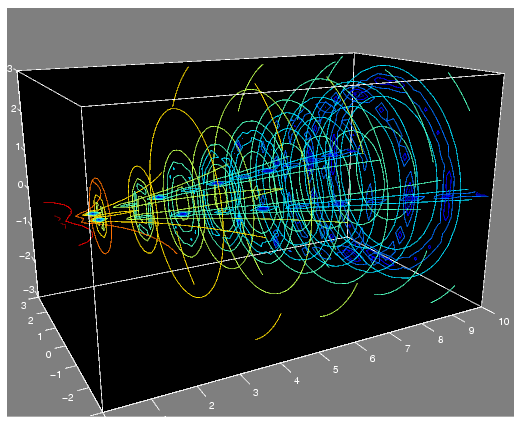MATLAB Function Referencecontourslice

Draw contours in volume slice planes

Syntax

• ```contourslice(X,Y,Z,V,Sx,Sy,Sz)
contourslice(X,Y,Z,V,Xi,Yi,Zi)
contourslice(V,Sx,Sy,Sz), contourslice(V,Xi,Yi,Zi)
contourslice(...,n)
contourslice(...,cvals)
contourslice(...,[cv cv])
contourslice(...,'`method`')
contourslice(axes_handle,...)
h = contourslice(...)
```

Description

`contourslice(X,Y,Z,V,Sx,Sy,Sz)` draws contours in the x-, y-, and z-axis aligned planes at the points in the vectors `Sx`, `Sy`, `Sz`. The arrays `X`, `Y`, and `Z` define the coordinates for the volume `V` and must be monotonic and 3-D plaid (such as the data produced by `meshgrid`). The color at each contour is determined by the volume `V`, which must be an m-by-n-by-p volume array.

`contourslice(X,Y,Z,V,Xi,Yi,Zi)` draws contours through the volume `V` along the surface defined by the 2-D arrays `Xi,Yi,Zi`. The surface should lie within the bounds of the volume.

`contourslice(V,Sx,Sy,Sz)` and `contourslice(V,Xi,Yi,Zi)` (omitting the `X`, `Y`, and `Z` arguments) assume `[X,Y,Z] = meshgrid(1:n,1:m,1:p)` where `[m,n,p]= size(v)`.

`contourslice(...,n)` draws `n` contour lines per plane, overriding the automatic value.

`contourslice(...,cvals)` draws `length(cval)` contour lines per plane at the values specified in vector `cvals`.

`contourslice(...,[cv cv])` computes a single contour per plane at the level `cv`.

`contourslice(...,'``method``')` specifies the interpolation method to use. `method` can be `linear`, `cubic`, or `nearest`. `nearest` is the default except when the contours are being drawn along the surface defined by `Xi`, `Yi`, `Zi`, in which case `linear` is the default (see `interp3` for a discussion of these interpolation methods).

```contourslice(axes_handle,...) ``` plots into the axes with handle `axes_handle` instead of the current axes (`gca`).

`h = contourslice(...)` returns a vector of handles to `patch` objects that are used to implement the contour lines.

Examples

This example uses the `flow` data set to illustrate the use of contoured slice planes (type `doc` `flow` for more information on this data set). Notice that this example

• Specifies a vector of `length = 9` for `Sx`, an empty vector for the `Sy`, and a scalar value (0) for `Sz`. This creates nine contour plots along the x direction in the y-z plane, and one in the x-y plane at z = 0.
• Uses `linspace` to define a ten-element vector of linearly spaced values from -8 to 2. This vector specifies that ten contour lines be drawn, one at each element of the vector.
• Defines the view and projection type (`camva`, `camproj`, `campos`).
• Sets figure (`gcf`) and axes (`gca`) characteristics.
• ```[x y z v] = flow;
h = contourslice(x,y,z,v,[1:9],[],,linspace(-8,2,10));
axis([0,10,-3,3,-3,3]); daspect([1,1,1])
camva(24); camproj perspective;
campos([-3,-15,5])
set(gcf,'Color',[.5,.5,.5],'Renderer','zbuffer')
set(gca,'Color','black','XColor','white', ...
'YColor','white','ZColor','white')
box on```

This example draws contour slices along a spherical surface within the volume.

• ```[x,y,z] = meshgrid(-2:.2:2,-2:.25:2,-2:.16:2);
v = x.*exp(-x.^2-y.^2-z.^2); % Create volume data
[xi,yi,zi] = sphere; % Plane to contour
contourslice(x,y,z,v,xi,yi,zi)
view(3)
```

See Also

`isosurface`, `slice`, `smooth3`, `subvolume`, `reducevolume`

Volume Visualization for related functionsContourgroup Properties contrast© 1994-2005 The MathWorks, Inc.